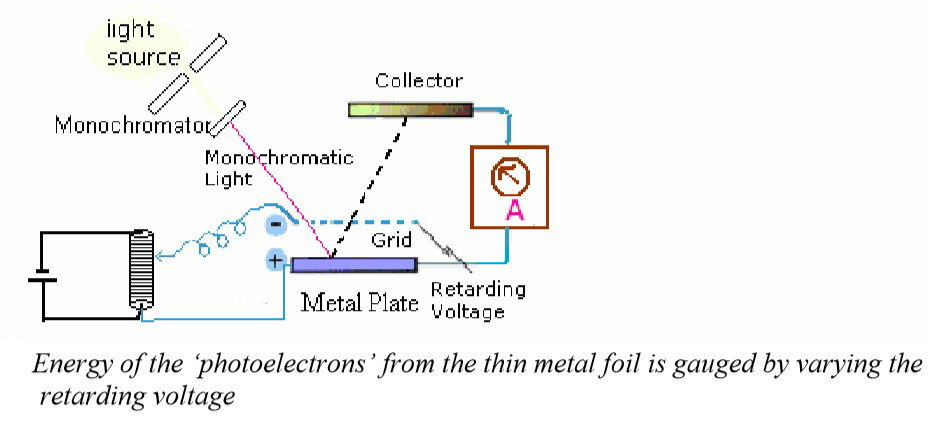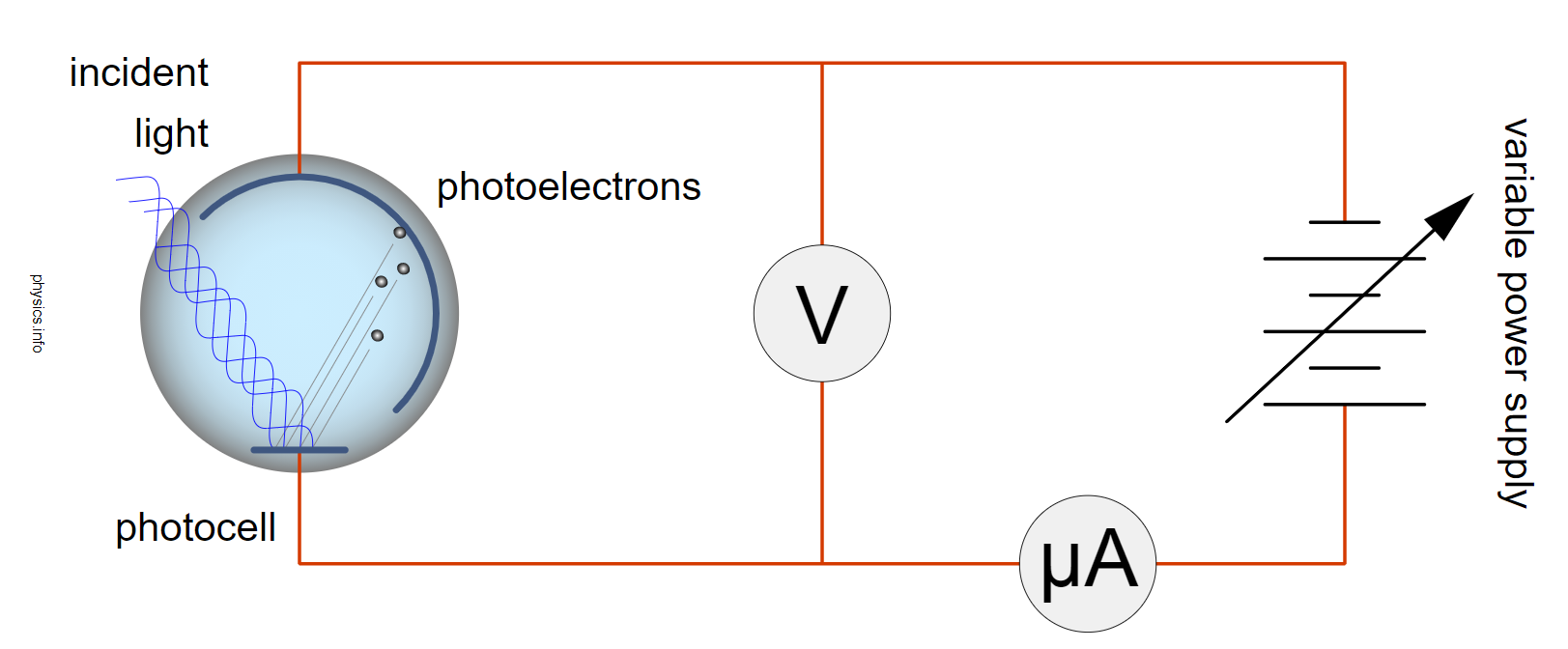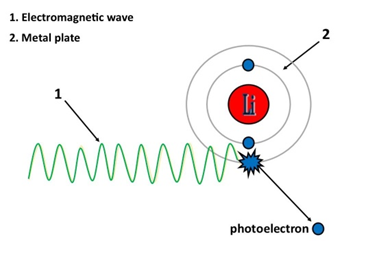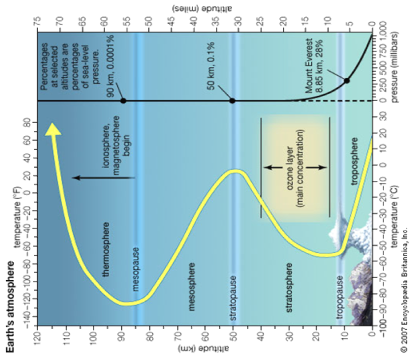It was in 1887 that the Photoelectric Effect was first observed by German physicist Heinrich Hertz. The theory of the Photoelectric Effect was further developed during the years 1886-1905 by Wilhelm Hallwachs and Philipp Lenard. They investigated the phenomenon of the photoelectric emission in detail and devised the experiment shown below. 

•Experiment devised by Philipp Lenard, Nobel prize winner 1905Schematic of experiment. 

In Lenard’s experiment, a power supply with an adjustable voltage is used to show  that it is the increasing frequency of the light (or one can refer to the light as an electromagnetic force), that induces an increasing current flow  reaction on the metal. There is a separation of electrons from the atoms of the metal, making positive ions and K.E electrons. This will happen with varying amounts of flow of electrons through the micro ammeter due to the frequency of the incident light applied. The incident light is an electromotive force (EMF) applied to the metal surface and Lenard found the greater the frequency of the EMF, would result in a greater frequency of electrons measured through the ammeter. The flow of electrons could be stopped by increasing the retarding voltage on the negative charged grid. This is because the increased negative voltage would stop the flow of the measured current due to the negative repulsion force being increased towards the photocell, and hence repulsing the negative electrons. Lenard suggests in this experiment how force measured by the frequency of light, has a curved linear relationship with the flow of electrons from a metal. Temperature change was not a consideration in the experiment set up, (and maybe not possible at this time in history due to technology requirements) and was not linked to a time parameter There was no observation possible to see the Endothermic Electric Effect reaction being caused by the emission of an electron from the atom.

What is an Electro Magnetic Force?

Force was first introduced in the modern era by Issac Newton in his famous book, ‘Principia Mathematica.’  Here Newton postulates,

"The law of universal gravitation, which he also confirmed from such further phenomena as the tides and the orbits of comets, states that every particle of matter in the universe attracts every other particle with a force that is proportional to the product of their masses and inversely proportional to the square of the distance between their centres."

Force = mass x acceleration

The Coulomb's law equation           

Coulombs law provides an accurate description of the force between two objects whenever the objects act as electric charges.

F = K (Q+ x q-) / R      (equation 4)

Where,

‘represents the Force generated between the two charges

‘is the Coulomb Constant which is equal to 8.99×109 N m2 C−2

Q+ and q- represent magnitude of charges

R‘ is the distance between the two charges

Since Coulomb's law applies to electric charges, the distance R in the equation is the distance between the centres of charge for both objects (not the distance between their nearest surfaces).

Q+ is the charged + ion sphere and interacts with q- due to attraction. The ion sphere has a positive charge from the centre of the ionised atoms and all q- charges are located from Q+ at a distance R in the growing negative part of the electric field.

Examples of forceFigure 5: A hand clap.

The sound wave produced is the result of the 2 objects or two operators (in this case 2 hands colliding). The sound wave produced is proof of the ‘need’ for two objects to produce force.

Hence for electricity to exist there must be ‘two operators’ or ‘two objects’ at work.figure 6: Photoelectric Effect .

The 2 objects of force in this example are,

1. Electromagnetic wave     2. Metal plate

It was Albert Einstein who was awarded the Nobel Prize for Physics in 1921 for ‘the Photoelectric Effect’. The honour was stated to be “for his services to ‘Theoretical Physics’, and especially for his discovery of the law of The Photoelectric Effect.” 

Einstein based his theory of the Photoelectric Effect as an incident electromagnetic energy being absorbed as a corpuscle of energy ‘hν’ by the atoms in the metal plate.

h = Planck’s Constant

v = Frequency

As a result, a part of the energy goes to the ‘work function’, φ ion+, the metal lattice, and part of the energy goes to the ejected electron in the form of ‘kinetic energy’ K.E.

The charge transferred to the metal atom ion and electron are given as: the opposite of each other and due to this relationship, we have two electric monopoles of attraction and repulsion, this is the fundamental idea of electromagnetism.

The energy applied as altered the state of the atoms and has not been diminished and is obeying the ‘Conservation of Energy’. Energy is transferred. Einstein expressed this, which is regarded as THE definition of ‘The Conservation of Energy’ in the following equation form:

E photon   = φ ion + KE electron

h ν = ion++ e-  (Equation 5)

Taking this logic and theory:

An EMF placed into the electric field charge of a lithium battery; we can write this equation:

Force = Li+ + e          (Equation 6)

Li+        = positively charged lithium atom ion

e-          = negatively charged lithium electron

Force   = applied EMF

The Photoelectric Effect is Parameter Based

• The photoelectric effect which is an electromagnetic force or electromagnetic wave and its observed reaction is parameter based,
• Hv (Planck’s constant and frequency)
• Density of metal
• Surface area
• hv, which has two components.
• ‘Planks constant         is a physical constant that is the quantum of electromagnetic action, which relates the energy carried by a photon to its frequency. A photon's energy is equal to its frequency multiplied by the Planck constant. The Planck constant is of fundamental importance in quantum mechanics, and in metrology it is the basis for the definition of the kilogram. The Planck constant is defined to have the exact value:
• h = 6.62607015×10−34 J⋅s            (Equation 7)       

### The first reaction seen in the thermosphere is ‘The photoelectric Effect’Figure 7: Earth’s atmosphere undertakes several charge states.

The first reaction seen in the thermosphere is ‘The photoelectric Effect’ and is the catalyst to an ‘Endothermic Electric Effect’ reaction as the atmosphere charges with heat energy from the top of the ionosphere to the ground. The reaction is first endothermic, then exothermic, it then becomes endothermic then exothermic again as seen. 

As seen in fig 7, the pressure of the system increases in respect to sea level pressure the further from the top of the ionosphere. I have on purposely placed fig 7 sideways as you can notice the similarity with the USW graph temperature curves.